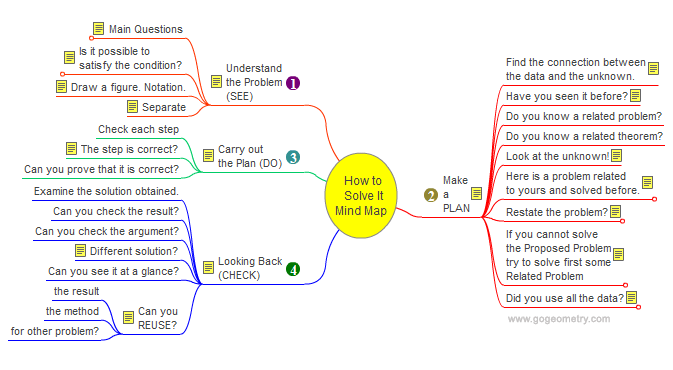Problem 693: Square, Inscribed and Circumscribed Circle, Areas, Mind Map, Polya. Level: High School, College, Math Ed. The figure shows a circumscribed circle about a square ABCD and the inscribed circle in the square ABCD. Prove that the area of the yellow shaded region is equal to the area of the blue shaded region.How to Solve It, Mind Map George Pólya's 1945 book "How to Solve It, A new aspect of Mathematical Method", is a book describing methods of problem solving. It suggests the following steps when solving a mathematical problem: (1) First, you have to understand the problem. (2) After understanding, then make a plan. (3) Carry out the plan. (4) Look back on your work. How could it be better?Recent Additions